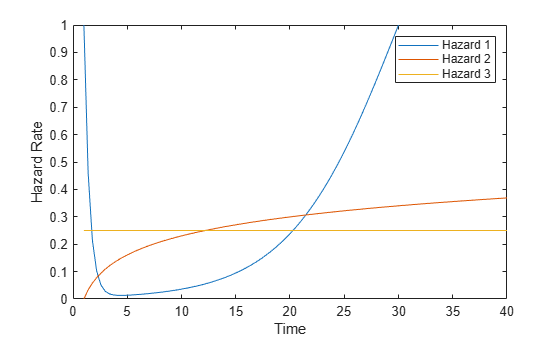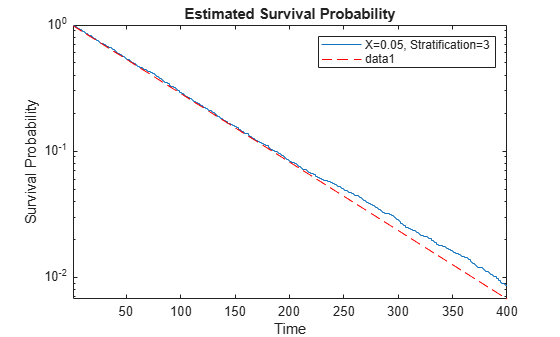# Cox Proportional Hazards Model Object

This example shows how to fit and analyze a Cox proportional hazards model using a `CoxModel` object. Cox proportional hazards models relate to lifetime or failure time data. For background, see What Is Survival Analysis? and Cox Proportional Hazards Model.

Generate the data for three lifetime models with the following types of hazard rates. These models correspond to three stratification levels; see Extension of Cox Proportional Hazards Model.

• Bathtub, whose failure rate is high at the beginning, decreases to a low level, then climbs toward a constant level

• Logarithmically increasing: $\mathrm{log}\left(x\right)/10$

• Constant $1/4$

The three models share a predictor with three multipliers for the base hazard rates:

• `1`

• `1/20`

• `1/100`

In total, the data has nine types of population members, one from each stratification level and one from each predictor level. The functions for creating the bathtub and logarithmic hazard rates are in the Helper Functions section at the end of this example.

Plot the three hazard rates when the predictor value is `1`.

```t = linspace(1,40); plot(t,hazard(t),t,log(t)/10,t,1/4*ones(size(t))) legend('Hazard 1','Hazard 2','Hazard 3','Location','northeast') ylim([0 1])```### Create Data for Fitting

Create pseudorandom data for the lifetimes associated with the nine models. Create 9000 samples chosen randomly (about 1000 of each type) by inverting the cumulative distributions. (For details, see Inverse transform sampling).

```N = 9000; rng default mults = [1;1/20;1/100]; % Three predictors strata = randi(3,N,1); % Three strata t1 = zeros(N,1); a0 = randi(3,N,1); % Predictor a1 = mults(a0); v1 = rand(N,1); for i = 1:N switch strata(i) case 1 % Bathtub t1(i) = zeropt(a1(i),v1(i)); case 2 % Logarithmic t1(i) = zeroptold(a1(i),v1(i)); case 3 % Constant t1(i) = 1 + exprnd(4/a1(i)); end end```

Place data into a table.

```a3 = categorical(a1); tbldata = table(t1,a3,strata,'VariableNames',["Lifetime" "Predictors" "Strata"]);```

### Fit Cox Model

Fit a Cox proportional hazards model to the table data.

`coxMdl = fitcox(tbldata,'Lifetime ~ Predictors',"Stratification",'Strata');`

### Plot Survival

Plot the probability of survival as a function of time for predictor value `1` and the three stratification levels.

```oo = categorical(1); plotSurvival(coxMdl,[oo;oo;oo],[1;2;3]) xlim([1,30])```Plot the probability of survival as a function of time for predictor value `1/20` and the three stratification levels.

```tt = categorical(1/20); figure plotSurvival(coxMdl,[tt;tt;tt],[1;2;3]) xlim([1,200])```Plot the probability of survival as a function of time for predictor value `1/100` and the three stratification levels.

```uu = categorical(1/100); figure plotSurvival(coxMdl,[uu;uu;uu],[1;2;3]) xlim([1,1000])```Even though the hazard rates are proportional for the different predictors, the three survival plots are not proportional.

### Analyze Fit

Examine the coefficients of the fitted model.

`disp(coxMdl.Coefficients)`
``` Beta SE zStat pValue ______ ________ ______ ______ Predictors_0.05 1.5301 0.031783 48.143 0 Predictors_1 4.5593 0.052149 87.427 0 ```

Notice that the `pValue` entries are `0`, which means that the listed predictor `Beta` values are not zero.

View the confidence intervals for the model coefficients at the 0.01 level of significance.

`coefci(coxMdl,0.01)`
```ans = 2×2 1.4483 1.6120 4.4249 4.6936 ```

To infer the hazard for the 0.01 level predictor, recall the definition of the Cox model:

$h\left({X}_{i},t\right)={h}_{0}\left(t\right)\mathrm{exp}\left(\sum \phantom{\rule{0.5em}{0ex}}{x}_{ij}{b}_{j}\right)$.

The `fitcox` function uses dummy variables with a reference group to handle categorical data. In this case, the function treats the 0.01 level predictor as the reference group, and encodes the predictor as all 0s when fitting the model. If you enter all 0s into the hazard function, you get

`$h\left(\left[0,0\right],t\right)={h}_{0}\left(t\right)\mathrm{exp}\left(0*b1+0*b2\right)={h}_{0}\left(t\right)\mathrm{exp}\left(0\right)={h}_{0}\left(t\right).$`

${h}_{0}\left(t\right)$ is the baseline hazard, which is stored in `coxMdl.Hazard`. Therefore, to get the hazard for the 0.01 level predictor, you can examine `coxMdl.Hazard`. Plot the baseline cumulative hazard for the three stratification levels.

```figure hold on for i = 1:3 pred3 = find(coxMdl.Hazard(:,3) == i); % Find indices of stratification i plot(coxMdl.Hazard(pred3,1),coxMdl.Hazard(pred3,2)) end xlabel('Time') ylabel('Cumulative Hazard') xlim([1 300]) legend('X = 0.01, Stratification 1',... 'X = 0.01, Stratification 2',... 'X = 0.01, Stratification 3','Location','northwest') hold off```The cumulative hazard for the other predictor values is $\mathrm{exp}\left(Beta\right)$ times the baseline cumulative hazard, where $Beta$ is the inferred coefficient.

`disp(exp(coxMdl.Coefficients.Beta))`
``` 4.6188 95.5127 ```

These relative hazard values are close to the theoretical values for the data, which was generated with multipliers 1, 1/20, and 1/100. The baseline value corresponds to the 1/100 multiplier, so the theoretical multipliers are 5 and 100.

View the `linhyptest` table.

`linhyptest(coxMdl)`
```ans=2×2 table Predictor pValue ___________________ ______ {'Empty Model' } 0 {'Predictors_0.05'} 0 ```

Again, the model requires the `1/20` value predictor and the 1 value predictor.

Check whether the data supports the hypothesis that the data is from a Cox proportional hazards model.

`supports = coxMdl.ProportionalHazardsPValueGlobal`
```supports = 0.9730 ```

The null hypothesis for this test is that the data is from a Cox proportional hazards model. To reject this hypothesis, `supports` must be less than 0.05 or some other small significance level. The statistic indicates support for the hypothesis that the data is consistent with a Cox model.

### Examine Hazard Ratios

Calculate the hazard ratio for the predictor values `1`, `1/20`, and `1/100` compared to a baseline of `0` for the three stratification levels.

```hazards = hazardratio(coxMdl,... categorical([1;1;1;1/20;1/20;1/20;1/100;1/100;1/100]),... [1;2;3;1;2;3;1;2;3],'Baseline',0)```
```hazards = 9×1 95.5127 95.5127 95.5127 4.6188 4.6188 4.6188 1.0000 1.0000 1.0000 ```

The hazard ratios are near their theoretical values of 100, 5, and 1, as explained in the previous section. The hazard ratios do not depend on the stratification level.

### How Well Does the Constant Hazard Stratification Level Match Theory?

Stratification level 3 has a constant hazard rate of 1/4. Theoretically, a constant hazard rate means an exponential survival function, whose logarithm is a straight line. Plot the survival of level 3 stratification using the predictor value `1/20`, which leads to an exponential rate of 1/80.

```tt = categorical(1/20); h = figure; axes1 = axes('Parent',h); plotSurvival(coxMdl,axes1,tt,3); xlim([1 400]); axes1.YScale = 'log'; hold on tms = linspace(1,400); semilogy(tms,exp(-tms/80),'r--') hold off```The data matches the theoretical line for probabilities well above 1/100.

### Helper Functions

This function creates the bathtub hazard rate.

```function h = hazard(x) h = exp(-2*(x - 1)) + (1 + tanh(x/10 - 3)); end```

This function creates the integral of the bathtub hazard rate from `1` to `x`.

```function eh = exphazard(x) eh = 1/2 - exp(-2*(x-1))/2; eh2 = (10*log(cosh(x/10 - 3)) - 10*log(cosh(1/10 - 3)) + x - 1); eh = eh + eh2; end```

This function solves for the root of the cumulative hazard rate with multiplier `a` to level `v`.

```function zz = zeropt(a,v) zz = fzero(@(x)(1 - exp(-a*exphazard(x)) - v),[1 100*max(1,1/a)]); end```

This function creates the integral of the logarithmic hazard rate with multiplier `1/10` from `1` to `x`.

```function cr = cumrisk(x) cr = 1/10*(x.*(log(x) - 1) + 1); end```

This function solves for the root of the cumulative hazard rate with multiplier `a` to level `v`.

```function zz = zeroptold(a,v) zz = fzero(@(x)(1 - exp(-a*cumrisk(x)) - v),[1 50*max(1,1/a)]); end```